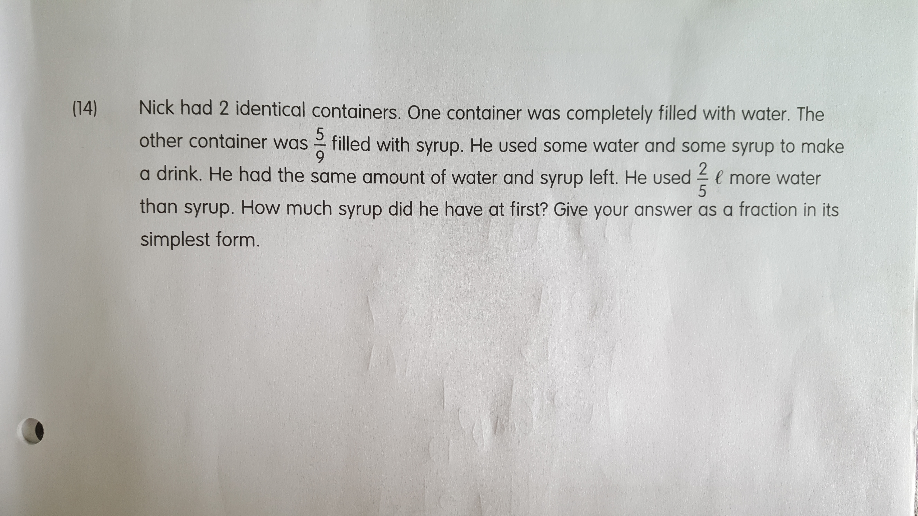# QuestionThis is a Before After question.

This question is the skill practice on the idea of Difference.

At before, there is a difference

9u – 5u = 4u

At after, there liquid are equal.

This means that the difference in  transfer (used) is the same.

4u = 2/5

1u = 2/5 ÷ 4 = 1/10

5u = 1/10 × 5 = 5/10 = 1/2 litres